Regression is a statistical relationship between two or more variables in which a change in the independent variable is associated with a change in the dependent variable. Logistic regression is used to estimate discrete values (usually binary values like 0 and 1) from a set of independent variables. It helps to predict the probability of an event by fitting data to a logistic function. This is called logistic regression.

 Want to master the advanced statistical concepts like linear and logistic regression? Check out the Data Science Course and learn today!

## Logistic Regression in R Tutorial

Logistic regression is kind of a misnomer in that when most people think of regression, they think of linear regression, which is a machine learning algorithm for continuous variables. However, logistic regression is a classification algorithm, not a continuous variable prediction algorithm.

This Logistic Regression in R article deals with the following topics:

• Why regression is used?
• What is regression?
• Types of regression
• Why logistic regression is used?
• What is logistic regression and how it works?
• Use-case implementation using logistic regression to predict college admission.

Let us begin by understanding: Why do we use regression?

## Why Regression?

Let’s say you have a website, and your revenue is based on the  website traffic, and you want to predict the revenue based on site traffic. The more traffic driven to your website, the higher your revenue would be, or at least that’s what you would intuitively assume.

In a plot of revenue versus website traffic, traffic would be considered the independent variable and revenue would be the dependent variable. The independent variable is often called the explanatory variable, and the dependent variable is called the response variable. However, they are typically referred to as independent and dependent variables. Our intuition tells us that the independent variable drives the dependent variable, and if there is some relationship between the two variables, then you would be able to use the independent variable to make predictions on the dependent variable.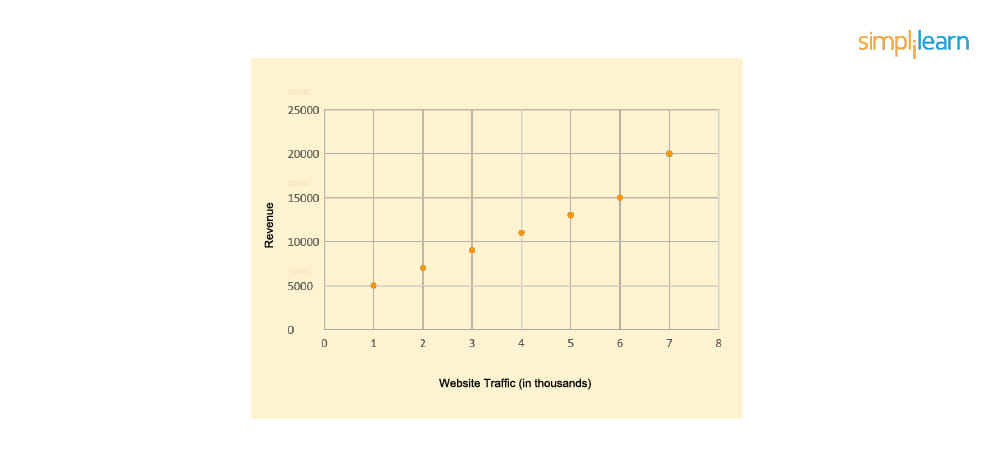This chart shows a clear trend between website traffic and revenue. As website traffic increases, the revenue increases. You can draw a line to show that relationship, and then you can use that line as a predictor line. So, for example, what will revenue be if your traffic is 4,500? If you draw a perpendicular line from 4.5K on the x-axis (the traffic axis) up to the orange regression line, sometimes called the line of best fit. Then you could draw another line over to the y-axis (the revenue axis) and see where it lands. You can see that when the traffic is around 4,500, the revenue is around 13,000.

Normally you wouldn’t actually draw those lines. You would generate an equation, and you would call that equation a model, and you could plug the independent variable into the equation to generate the dependent variable output, which you would call your prediction.

## What Is Regression?

Regression is a statistical relationship between two or more variables in which a change in the independent variable is associated with a change in the dependent variable.It’s important to note that not all variables are related to each other. For example, a person’s favorite color may not be related to revenue from a website. But if you look at a chart showing height and age, the change in one variable—height—is closely associated with the change in the other variable—age. This makes intuitive sense, as from birth, as you get older, you get taller. If you plot that data, you would see those green points on the graph up to some certain age where growth would taper off. The plot in the middle shows the clear linear relationship between age and height, which is indicated by the solid red line. You sometimes call that line a trendline, or a regression line, or the line of best fit. You see that the height is the dependent variable, and age is the independent variable.

You might ask, “Doesn’t height depend on other factors?” Of course it does, but here we’re looking at the relationship between two variables, one independent and one dependent: age and height.

Next, let us take a look at the types of regression.

## Types of Regression

There are various types of regression:

• Linear regression logistic
• Logistic regression
• Polynomial regression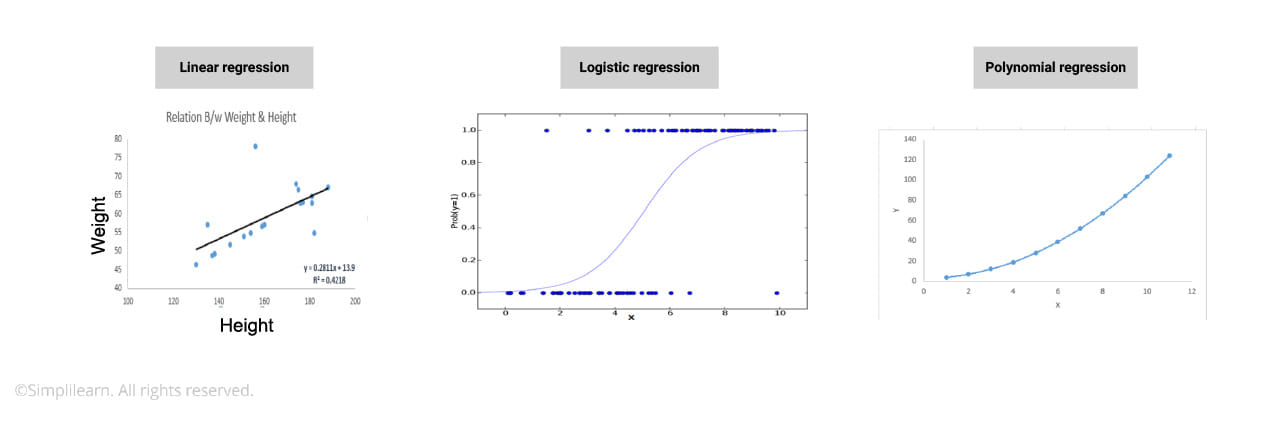Linear regression is probably the most well known. By definition, when there is a linear relationship between a dependent variable—which is continuous—and an independent variable—which is continuous or discrete—you would use linear regression.

When the Y value in the graph is categorical—such as yes or no, true or false, the subject did or did not do something—then you would use logistic regression. Logistic regression is when the Y value on the graph is categorical in nature and depends on the X variable. Notice that the trendline for linear regression and the line for logistic regression are different—more on that later.

Polynomial regression is when the relation between the dependent variable Y and the independent variable X is in the nth degree of X. In a plot, you can see that the relationship is not linear; there’s a curve to that best-fit trendline.

## Why Logistic Regression?

You need to understand why you would use logistic regression and not linear regression. Picking the machine learning algorithm for your problem is no small task. It really behooves you to understand linear regression vs. logistic regression.

Linear regression answers the question, “How much?” In our earlier example, as website traffic grows, how much will revenue grow?

Whereas logistic regression predicts if something will happen or not happen. Linear regression is generally used to predict a continuous variable, like height and weight. Logistic regression is used when a response variable has only two outcomes: yes or no, true or false.

We refer to logistic regression as a binary classifier, since there are only two outcomes. Let’s try to understand this with an example. Let’s say you have a startup company and you are trying to figure out whether the startup will be profitable or not. That’s binary, with two possible outcomes: profitable or not profitable. So let’s use initial funding to be the independent variable.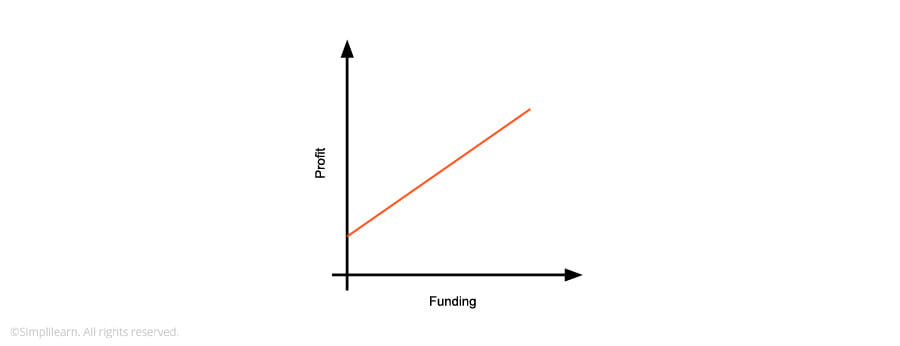This graph shows funding versus profit, and it appears linear. Once again, our intuition tells us that the more funding a startup has, the more profitable it will be, but of course data science doesn’t depend on intuition; it depends on data.

This graph does not tell whether the startup will be profitable or not; it states only that with an increase in funding, the profit also increases. That’s not binary. If you want to predict how much profit will be made, linear regression would be useful, but that’s not what you are trying to figure out here. Hence you need to make use of logistic regression, which is two outcomes—in our case, profitable and not profitable.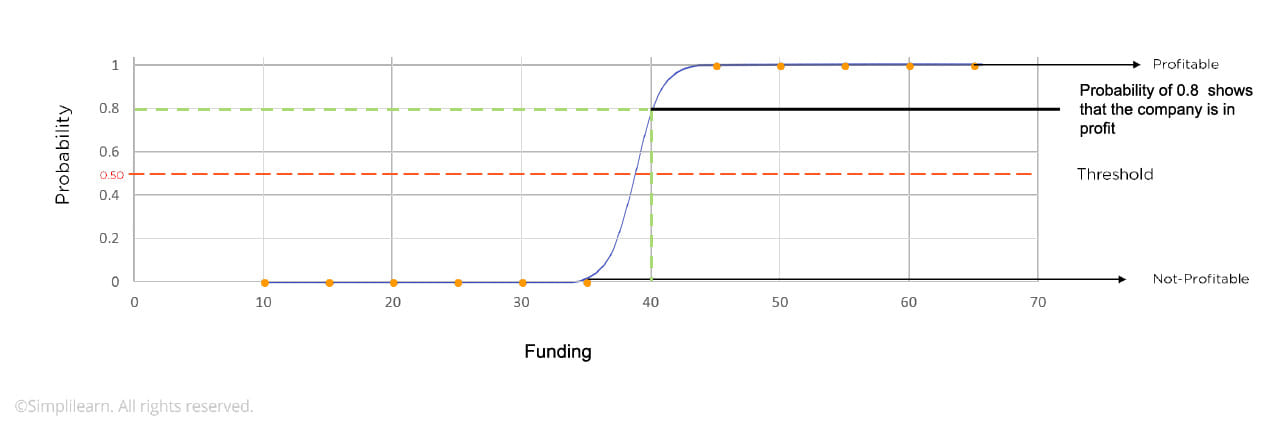In the next graph, the x-axis is our independent variable, funding. The y-axis is no longer the dependent variable, profit, but rather the probability of profit. For example, if you look at a company with a funding of, say, 40, then the probability  that the company will be profitable is around 0.8 or 80 percent, based on the best-fit line, called a sigmoid curve.

In the example, we plotted several companies with various funding levels from 10 to 70 and  indicated whether they were zero—not profitable—or 1—profitable—on the graph. This is how you should think of logistic regression.

In this example, given the amount of funding, we can calculate the probability that a company will be profitable or not profitable. If you use the threshold line of 0.5, then you have your classifier. If the probability is 0.5 or higher, the company is profitable; if the probability is lower than 0.5, it’s not profitable.

## What Is Logistic Regression?

Let’s compare linear regression to logistic regression and take a look at the trendline that describes the model.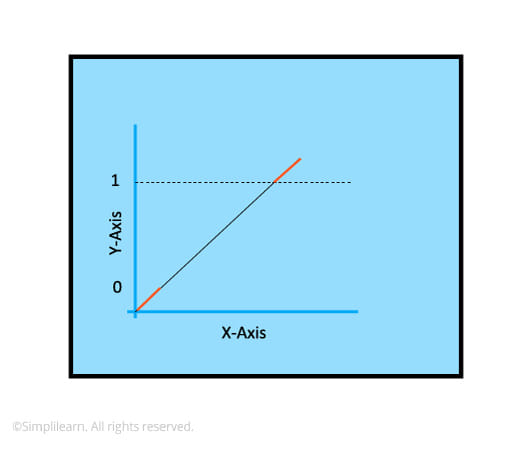In the linear regression graph above, the trendline is a straight line, which is why you call it linear regression. However, using linear regression, you can’t really divide the output into two distinct categories—yes or no—to divide our results into two categories. You would have to clip the line between 0 and 1. If you recall, probabilities can be between only 0 and 1, and if we’re going to use probability on the y-axis, then you can’t have anything that is below 0 or above 1.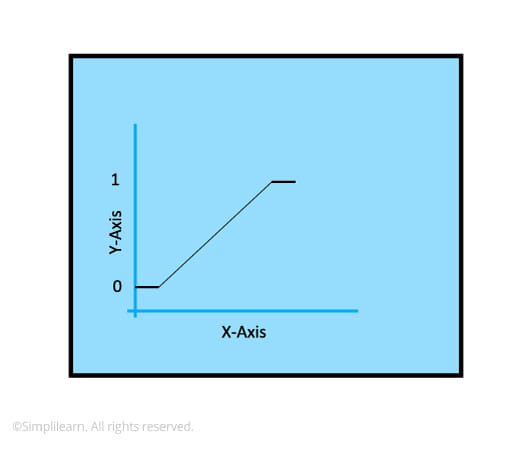Thus you would have to clip the line, and once you clip the line, you see that the resulting curve cannot be represented in a linear equation.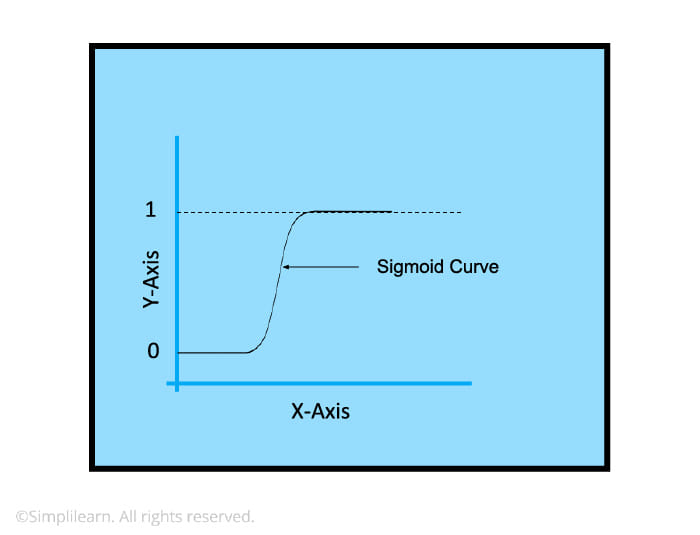For logistic regression, you will make use of a sigmoid function, and the sigmoid curve is the line of best fit. Notice that it’s not linear, but it does satisfy our requirement of using a single line that does not need to be clipped.

For linear regression, you would use an equation of a straight line:

y = b0 + b1*x,

where x is the independent variable, y is the dependent variable.

Because you cannot use a linear equation for binary predictions, you need to use the sigmoid function, which is represented by the equation:

p = 1/(1+e-y)

e is the base of the natural logs.

Then by taking the log of both sides and solving it, you get the sigmoid function. By graphing it, you get the logistic regression line of best fit.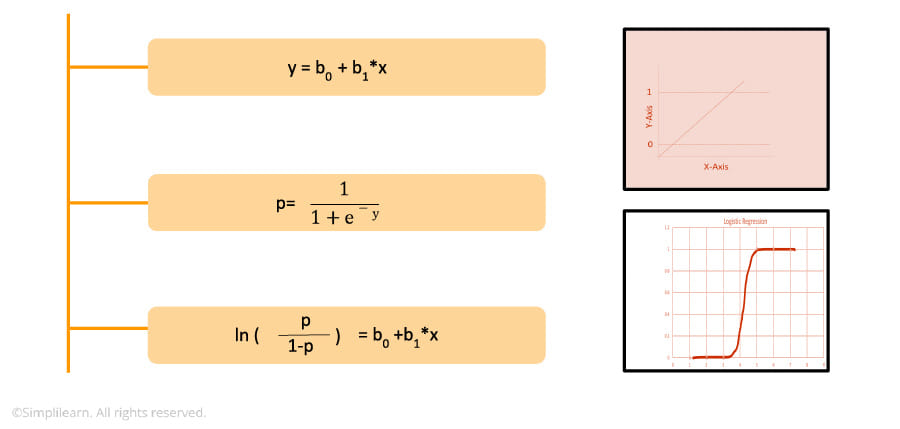Next, let us get more clarity on Logistic Regression in R with an example.

### Logistic Regression Example: College Admission

The problem statement is simple. You are given a dataset, and you need to predict whether a candidate will get admission in a desired college or not, based on the person’s GPA and college rank.

It’s important to note that in the dataset that we’ve imported, we were given the GPAs and college ranks for several students, but it also has a column that indicates whether those students were admitted or not. Based on this labeled data, you can train the model, validate it and then use it to predict the admission for any GPA and college rank. Once you split the data into training and test sets, you will apply the regression on the two independent variables (GPA and rank), generate the model and then run the test set through the model. Once that is complete, you will validate the model to see how well it performed.

Here is the video that represents the steps followed to implement the use case.

The very first thing you need to do is import the data set that you were given in CSV format (comma-separated values). Next, select and import the libraries that you will need. Although R is a great programming language with a lot of built-in functions, it is easily and powerfully extended by the use of libraries and packages. Then you need to split the data set into a training set and a test set.

After the library is loaded, you set your working directory. In that working directory, there’s a file called binary dot CSV, and that’s the CSV file from the college. In this case, the data has four columns: GRE, GPA rank and then the answer column: whether or not someone was admitted (value = 1) or not admitted (value = 0).

Now it’s time to split the data. Take the data frame and split it into two groups, a training set and a test set. The demo uses an 80/20 ratio, so 80 percent of the data will go into the training set and 20 percent will go into the test set. Of course that ratio could be 60/40 or 70/30. It really depends on the size of your data, but in our example and for our purposes, 80/20 is perfect. Next, we’ll do a little data munging. In general you munge the data early on after ingestion, and you really have to be careful. In this case you don’t have any missing values; you don’t have any real outliers. Our data was pretty clean when we got it and ingested it, but in general that’s not the case, and you need to put in a lot of work and pay a lot of attention to the munging process here.

 How good are your skills in Logistic Regression? Take up this Data Science test and find out today!

We’re going to use the GLM function (the general linear model function) to train our logistic regression model and the dependent variable. The independent variables are GPA and rank, and a little tilde sign here says the dependent variable will be a function of GPA and rank. The two independent variables in the data will be the training set, and the family will be binomial; binomial indicates that it’s a binary classifier. It’s a logistic regression problem.

There it is: You ran your model, and there’s a summary of your model. You can see that there is some statistical significance in GPA and in rank by the coefficients and output of the model. So next let’s run the test data through the model. Next, set up a confusion matrix and look at your predictions versus the actual values. Again, this is important. You had the answers and you predicted some answers, so hopefully our predicted answers match up with the actual answers.

To check that, run a confusion matrix so you can see the predicted values versus the actual values. It’s important here to know if it was predicted false and it was actually false, or if it was predicted true and it was actually true.

Logistic regression is a binary classifier, and it’s very good at that in general. Are there other binary classifiers? Yes, but logistic regression is easy to understand and easy to implement, and that means it’s often the first choice.

## What Next?

Logistic Regression is one of the important concepts when dealing with data analytics using R programming language. But, why should you restrict yourself by understanding only a small portion of data analytics? You can master various other concepts like data visualization, data exploration, predictive analytics and descriptive analytics techniques with the R language by taking Simplilearn’s Data Science Certification Training - R Programming. This data science course is an ideal package that you can take to become a successful data analyst. So get started and become certified!

## About the AuthorSimplilearn

Simplilearn is one of the world’s leading providers of online training for Digital Marketing, Cloud Computing, Project Management, Data Science, IT, Software Development, and many other emerging technologies. Based in San Francisco, California, and Bangalore, India, Simplilearn has helped more than 500,000 students, professionals and companies across 200 countries get trained, upskilled, and acquire certifications.

View More
• Disclaimer
• PMP, PMI, PMBOK, CAPM, PgMP, PfMP, ACP, PBA, RMP, SP, and OPM3 are registered marks of the Project Management Institute, Inc.